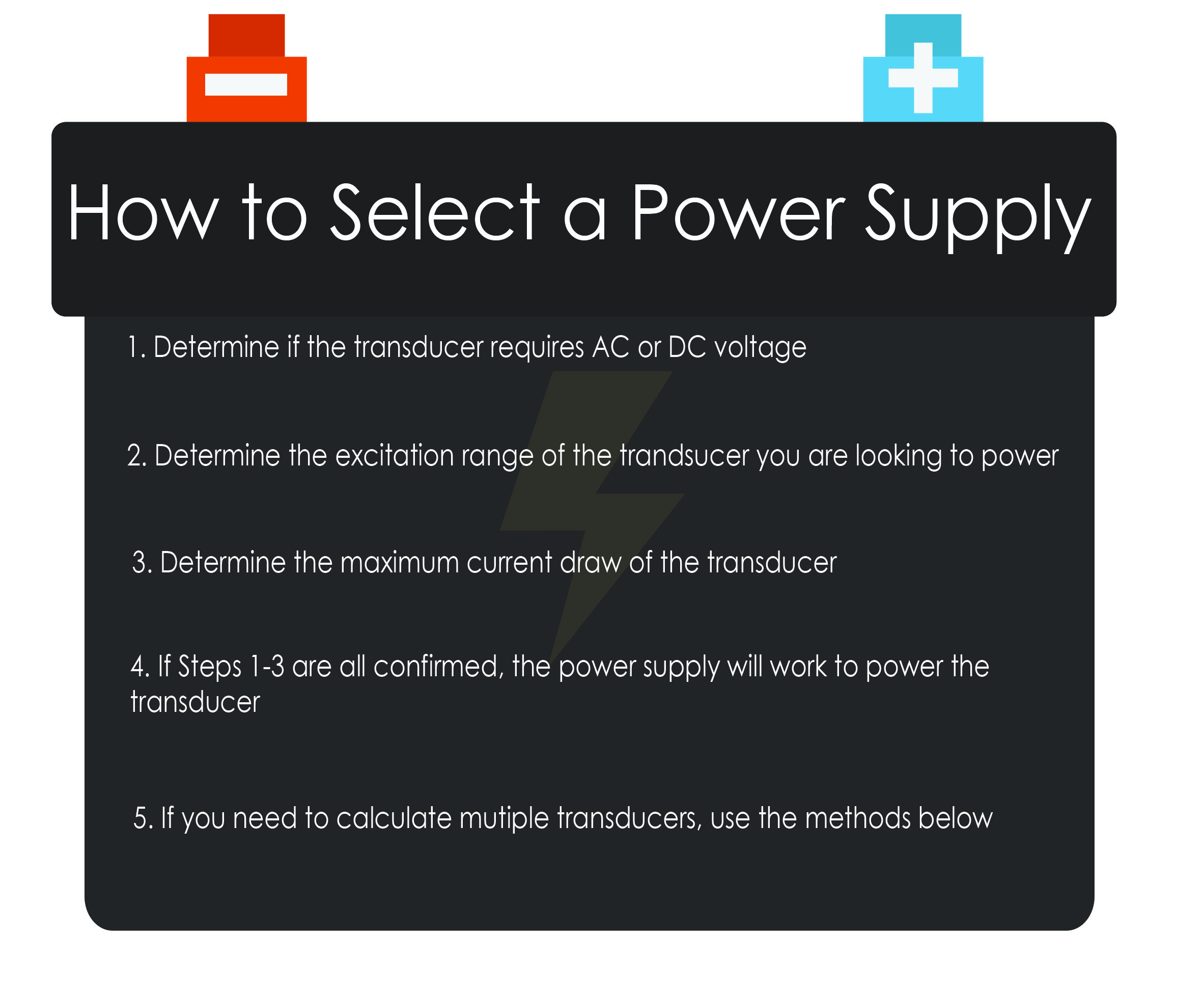### Scales

July 25, 2016

#### by Jeremy Bibeau

After selecting your pressure transducer, it is now time to select a power supply. It is important to select the proper power supply to ensure all components are functioning properly and recording accurate data.How To Calculate Multiple Transducers

Method 1:  Take the sum of the current draws of all the transducers and verify that the amount is less than or equal to the total current output of the power supply

• For example, if you have a power supply of 100 mA and three pressure transducers consuming 25 mA, 50 mA, and 50 mA, you would not be able to power all three pressure transducers using the same power supply. Since the sum of the current draw (25 mA+ 50 mA+ 50 mA= 125 mA) exceeds the current amount the power supply can provide, you would need to select an additional power supply. However, the power supply can be configured with two transducers since the sum will not exceed the output of the supply source

Method 2: If each pressure transducer consumes the same amount of current divide the max current output of the power supply by the power consumption of the pressure transducer to determine how many transducers can be powered

• For example, if you have a power supply of 100 mA and a pressure transducer requiring 25 mA, you can power a total of four pressure transducers ((100/25)=4) requiring 25 mA current